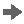###Exchange Rate Spread

#### Definition

Constant difference between the average rate and the buying rate, or between the average rate and the bank selling rate.

#### Use

For exchange rate types, you can define fixed exchange rate spreads between average rate and buying rate, as well as between average rate and bank selling rate.

You then only have to enter exchange rates for the average rate. The system then calculates the exchange rates for the buying rate and bank selling rate by adding and subtracting the exchange rate spread for the average rate.Recommendation

SAP recommends defining a reference currency for the average rate and then entering exchange rate spreads for the buying and bank selling rates. This combination is particularly efficient since you only have to enter exchange rates for the individual currencies to the reference currency for the average rate exchange rate type. The system calculates all the other exchange rates.

End of the recommendation.